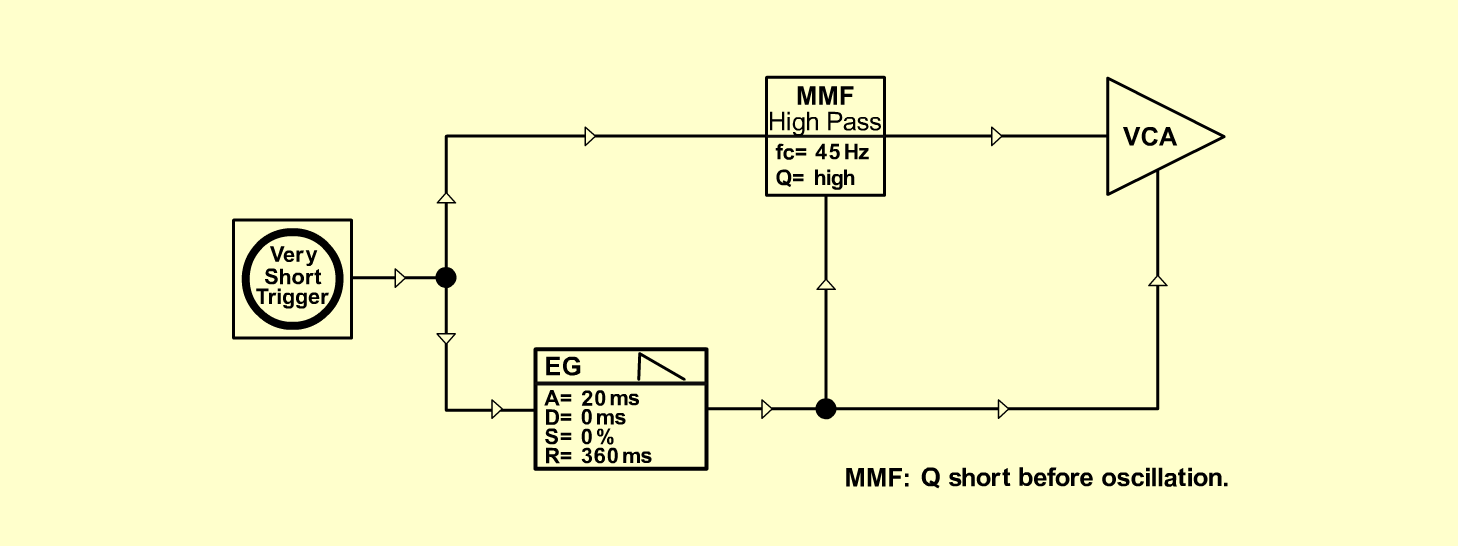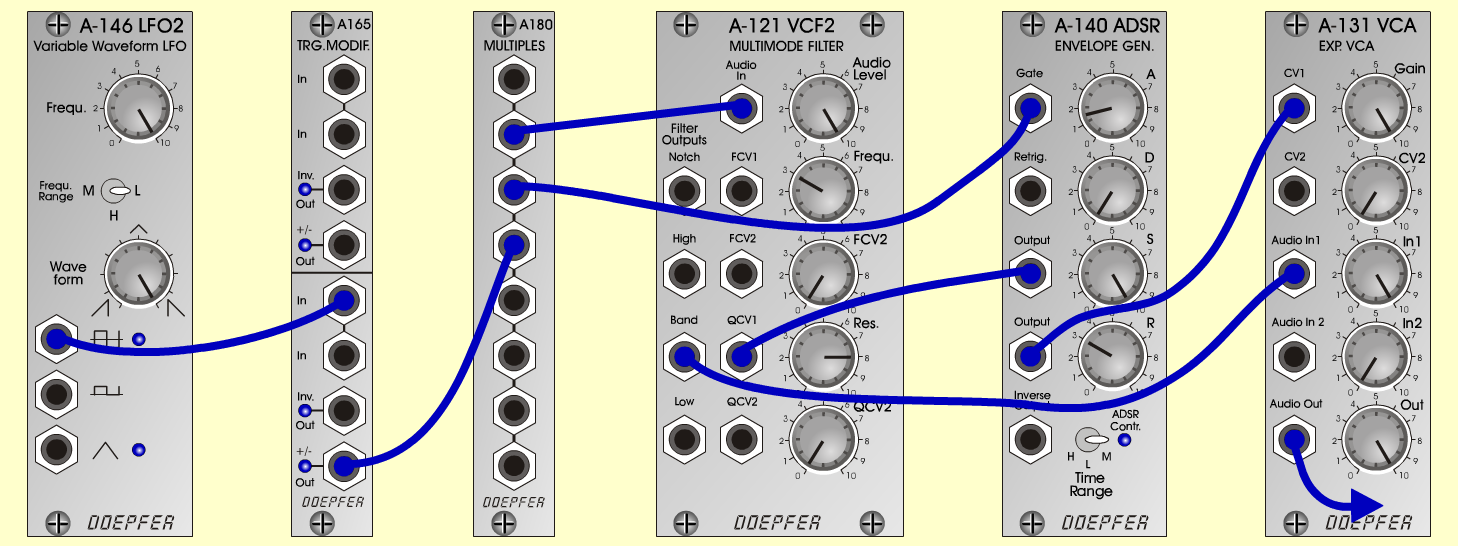Bass DrumD r u m sD o e p f e r A - 100 Connections: Settings: A-121 (Band)  <=>  A-131 (Audio In)   A-140 (Output 1)  <=>  A-121 (QCV 1)   A-140 (Output 2)  <=>  A-131 (CV 1)   A very short trigger: KB, Seq or   A-146 (Square)  <=>  A-165 (In)   A-165 (+/- Out)  <=>  A-180   A-180  <=>  A-121 (Audio In)   A-180  <=>  A-140 (Gate) A-146 (Freq = 10, Wave Form = 10, Range = L)   A-121 (Audio Level = 10, Freq = 3, Res = 8)   A-140 (A = 1.5, D = 0, S = 10, R = 3, Range = M)   A-131 (Gain = 10, Audio In 1 = 10,   Audio Out = 10) Notes: Set Q from A-121 short before oscillation. Josef MuellerSound sample BassDrum